In this post I will demonstrate how to integrate database into Flask. I will build a small form containing text input. This input is dynamically populated through `ajax` when you start typing things. The data that ajax populates is coming from postgresql database. The purpose of this post is to document things for my future usage. However, I will try to inject comments and guides for you if you want to replicate or use some of these techniques. I can not guarantee that my methods used here are the best. In fact, as I am learning these things myself, there are always better ways to do things, and I hope you can comment on some of them.

As I have written earlier post on Flask, here I will concentrate on the three concepts not used earlier: Form, SQLAlchemy and Ajax.

## Form

I am using the flask extension called `flask_wtf` and `WTForms`. First, `pip install Flask-WTF WTForms`. In your `app.py` import them.

``````from flask_wtf import FlaskForm
from wtforms import StringField
from wtforms.validators import DataRequired, Length
``````

Above, I have imported `StringField` and few validators.

You have to add the following two `config` stuff.

``````app.config['WTF_CSRF_ENABLED'] = True
app.config['SECRET_KEY']='my_love_dont_try'
``````

Now, create the form class.

``````class MyForm(FlaskForm):
country = StringField('Country', validators=[DataRequired(),
Length(max=40)],render_kw={"placeholder": "country"})
``````

Let us build the template that uses this form. I will call it `sdg.html`.

``````<form class="form-inline">
{{ form.csrf_token }}
<div class="form-group">
{{form.country(class="form-control")}}
</div>
<button type="submit" class="btn btn-info">Submit</button>
</form>
<div id="result"></div>
``````

Note the use of `csrf_token` inside the form. Also, note how I was able to pass `CSS` class names to the form. You may recognize these `bootstrap 4` classes. The `<div id='result'>` will be place holder to hold result of processing the form later.

Finally, let us build the route function to serve this form in our `app.py`

``````@app.route('/form')
def sdg():
form = MyForm()
return render_template('sdg.html', form=form)
``````

Here is how it looks.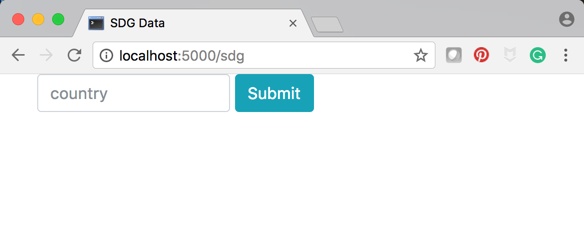## SQLAlchemy

I am using `flask_sqlalchemy`. So, first `pip install` it and then import it

``````from flask_sqlalchemy import SQLAlchemy
``````

Next, you need to insert the following `config` parameter to specify the connection string. I am using `postgresql` database.

``````app.config['SQLALCHEMY_DATABASE_URI']= 'postgresql://localhost/sdg'
``````

You need next to instantiate.

``````db = SQLAlchemy(app)
``````

My database model contains information about countries an has two fields: name of the country and the 3-letter iso code of the country as follows.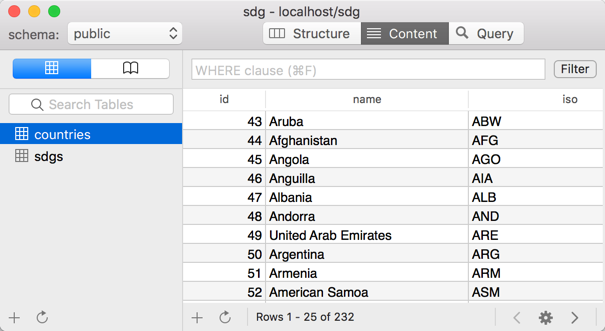So, let us define this model.

``````class Country(db.Model):
__tablename__ = 'countries'

id = db.Column(db.Integer, primary_key=True)
name = db.Column(db.String(60), unique=True, nullable = False)
iso = db.Column(db.String(3), unique=True, nullable = False)

def __repr__(self):

return '{} - {}'.format(self.iso, self.name)

def as_dict(self):
return {'name': self.name}
``````

The function `as_dict` is a handy function to allow producing a dictionary out of this country model. This proves to be helpful when trying to use list of countries inside jsonify function for example.

I assume that you already created this database table in `postgres` in a way or other.

Now, let us test this table. I will create a route function that would return all countries in a json format as follows

``````@app.route('/countries')
def countrydic():
res = Country.query.all()
list_countries = [r.as_dict() for r in res]
return jsonify(list_countries)
``````

Note, how I was able to create a list of dictionaries because of the `as_dict()` function.

Here is the result.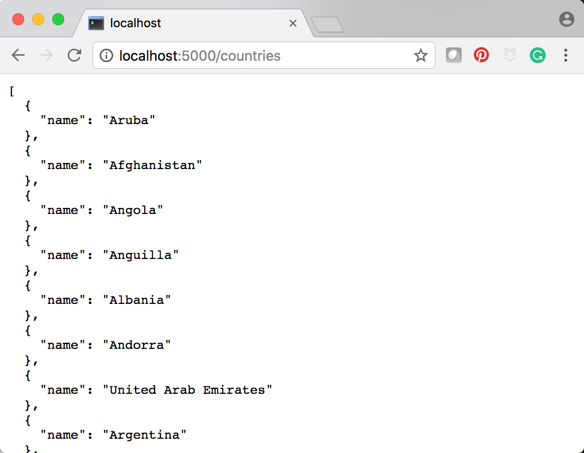## Jquery and Ajax

My intention is that whenever I start typing names in the country field, `jquery` should automatically populate the filed with names of countries.

``````\$(document).ready(function(){
var countries=[];

\$.getJSON('/countries', function(data, status, xhr){
for (var i = 0; i < data.length; i++ ) {
countries.push(data[i].name);
}
});
};

\$('#country').autocomplete({
source: countries,
});
});
``````

The function `loadCountries` retrieve the json dictionary from the url `/countries` and populate the array `countries`. All that is left is to use the `jquery` function `autocomplete`.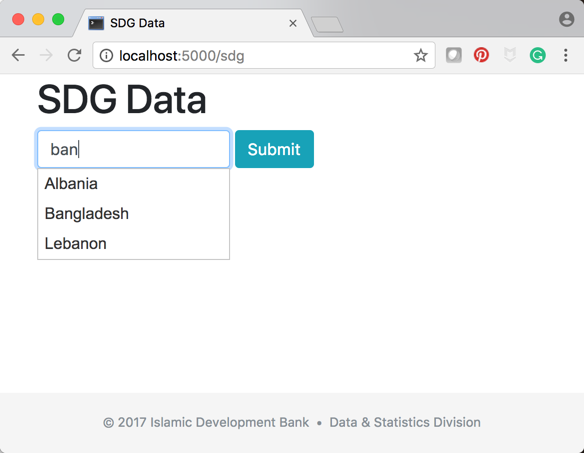Finally, for the sake of completeness, let us process the form, again through ajax technology. To be super simple, I will just place whatever is the input from country field into the result `div`.

For, this I write the following `on('submit')` function.

``````\$('form').on('submit', function(e){
\$.ajax({
data: {
country:\$('#country').val()
},
type: 'POST',
url : '/process'
})
.done(function(data1){
if (data.error){
\$('#result').text(data.error).show();
}
else {
\$('#result').html(data.country).show()
}
})

e.preventDefault();
});
``````

In my `app.py` I need to provide the `/process` function as follows:

``````@app.route('/process', methods=['POST'])
def process():
country = request.form['country']
if country:
return jsonify({'country':country})
return jsonify({'error': 'missing data..'})
``````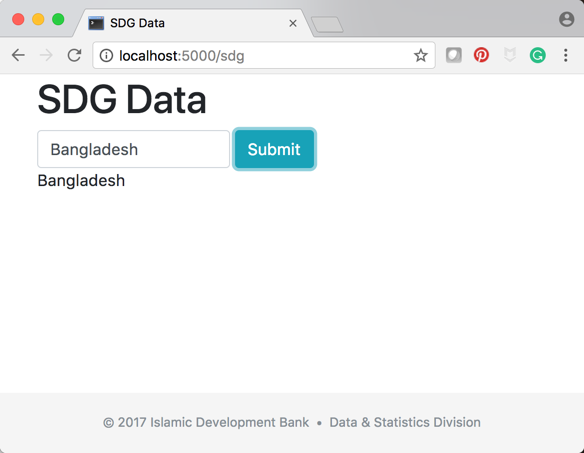Finally, you can find the project in this github repository.